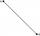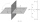Right triangle from axes

A line segment has its ends on the coordinate axes and forms with them a triangle of area equal to 36 sq. Units . The segment passes through the point ( 5,2). What is the slope of the line segment. ?

Result

k1 =  -0.015
k2 =  -10.705

Solution:Checkout calculation with our calculator of quadratic equations.Leave us a comment of example and its solution (i.e. if it is still somewhat unclear...):Be the first to comment!To solve this verbal math problem are needed these knowledge from mathematics:

For Basic calculations in analytic geometry is helpful line slope calculator. From coordinates of two points in the plane it calculate slope, normal and parametric line equation(s), slope, directional angle, direction vector, the length of segment, intersections the coordinate axes etc. Looking for help with calculating roots of a quadratic equation? Need help calculate sum, simplify or multiply fractions? Try our fraction calculator. Pythagorean theorem is the base for the right triangle calculator.

Next similar examples:

1. On lineOn line p: x = 4 + t, y = 3 + 2t, t is R, find point C, which has the same distance from points A [1,2] and B [-1,0].
2. Sphere equationObtain the equation of sphere its centre on the line 3x+2z=0=4x-5y and passes through the points (0,-2,-4) and (2,-1,1).
3. Circle annulusThere are 2 concentric circles in the figure. Chord of larger circle 10 cm long is tangent to the smaller circle. What are does annulus have?
4. SegmentCalculate the length of the segment AB, if the coordinates of the end vertices are A[10, -4] and B[5, 5].
5. RT and circlesSolve right triangle if the radius of inscribed circle is r=9 and radius of circumscribed circle is R=23.
6. CathetiThe hypotenuse of a right triangle is 41 and the sum of legs is 49. Calculate the length of its legs.
7. Symmetry by planeDetermine the coordinates of a image of point A (3, -4, -6) at a symmetry that is determined by the plane x-y-4z-13 = 0
8. Parametric equationFind the parametric equation of a line with y-intercept (0,-4) and a slope of -2.
9. Circle - AGFind the coordinates of circle and its diameter if its equation is: ?
10. Theorem proveWe want to prove the sentence: If the natural number n is divisible by six, then n is divisible by three. From what assumption we started?
11. RectangleCalculate area of the rectangle if its length is 12 cm longer than its width and length is equal to the square of its width.
12. Equation of circlefind an equation of the circle with indicated properties: a. center at (-3,5), diameter 20. b. center at origin and diameter 16.
13. RootsDetermine the quadratic equation absolute coefficient q, that the equation has a real double root and the root x calculate: ?
14. DiscriminantDetermine the discriminant of the equation: ?Find the roots of the quadratic equation: 3x2-4x + (-4) = 0.Equation ? has one root x1 = 8. Determine the coefficient b and the second root x2.The lengths of a parallel sides of a trapezium are (2x+3) and (x+8) and the distance between them is (x+4). if the area of the trapezium is 590 , find the value of x.